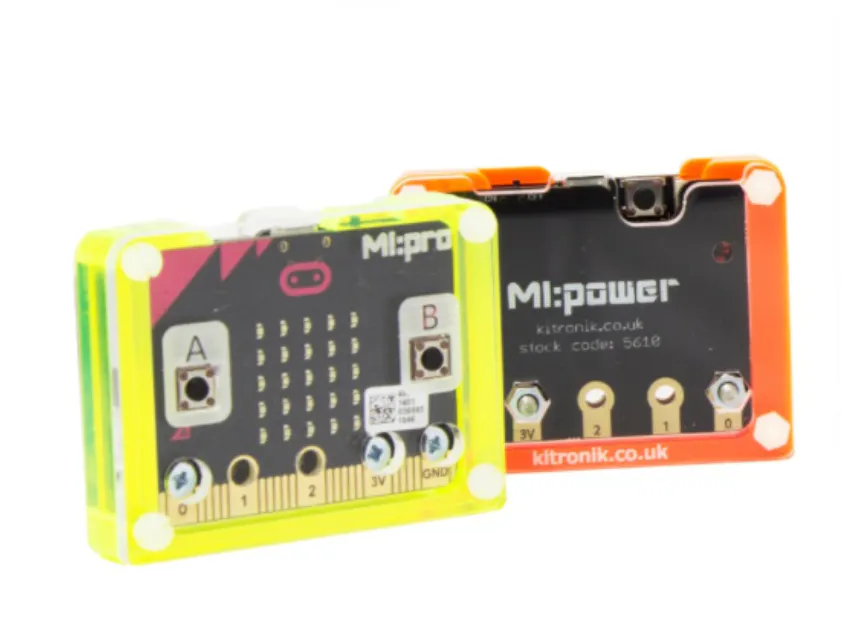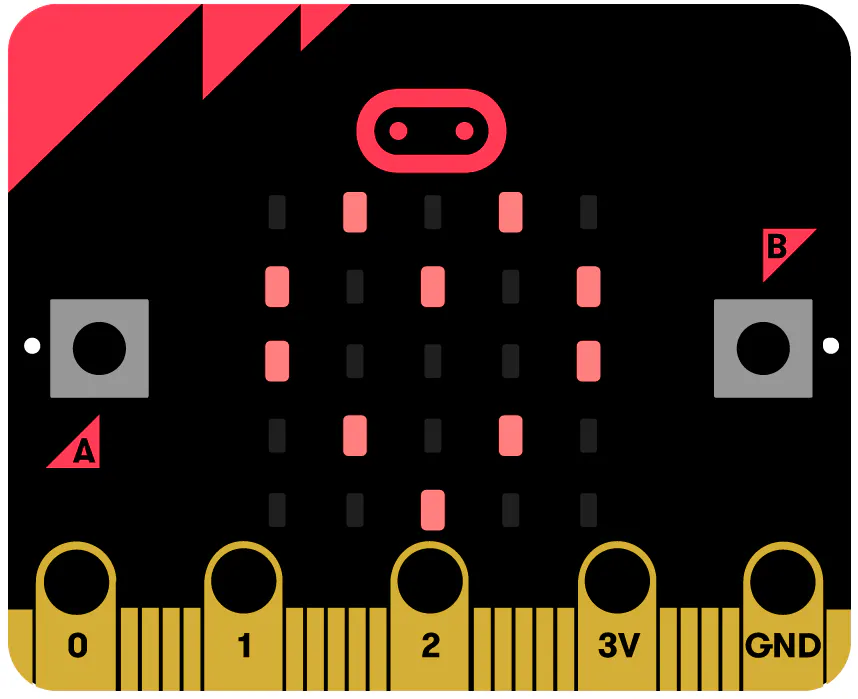Published

# Math Training Thru Micro:bit Icons (ClientVersion)

A Micro:bit-based game to help teachers train their students in math through a fun and competitive manner by counting LEDs in icons.

IntermediateFull instructions provided1 hour133## Things used in this project

### Hardware componentsBBC micro:bit board
×1
 Kitronik MI:Power Board
×1
 Kitronik MI:pro Protector Case
×1

Read more

## Code

### A Microbit-Based Competitive Math Training Game Using the Counting of Icon LEDs (Client Version)

JavaScript
It's a Math teacher's tool for training her students in math through a fun and competitive manner.
```let Z = 0
let Y = 0
let Img3: Image = null
let X = 0
let Img2: Image = null
let WinSw = 0
let PressButtB = 0
let Img1: Image = null
let PressButtA = 0
let B1 = 0
let ShowCorrSw = 0
let MyDeviceNo = 0
let A1 = 0
let RadioAns = 0
let Wins = 0
let IconsOld = 0
let IctrLst: number[] = []
let RadioRes = 0
let PressOthButt = 0
let PressOthButtB = 0
let GotCorrect = 0
let IconLst: Image[] = []
let final_answer = 0
let Icons = 0
radio.onDataPacketReceived( ({ receivedString: name, receivedNumber: value, serial: serial2 }) =>  {
if (name == "ICON") {
Icons = value
if (Icons != IconsOld) {
IconsOld = Icons
ShowCorrSw = 0
GotCorrect = 0
A1 = 0
B1 = 0
WinSw = 0
RadioAns = 0
RadioRes = 0
PressButtA = 0
PressButtB = 0
PressOthButt = 0
PressOthButtB = 0
final_answer = 0
Y = value % 10000
Z = Y % 100
X = value - Y
X = X / 10000
Y = Y - Z
Y = Y / 100
X += -10
Y += -10
Z += -10
Img1 = IconLst[X]
Img2 = IconLst[Y]
Img3 = IconLst[Z]
for (let i = 0; i < 3; i++) {
basic.clearScreen()
}
Img1.showImage(0)
basic.pause(1000)
Img2.showImage(0)
basic.pause(1000)
Img3.showImage(0)
basic.pause(1000)
basic.clearScreen()
}
} else {
if (name == "IID") {
if (value == MyDeviceNo) {
if (GotCorrect == 0) {
GotCorrect = 1
if (ShowCorrSw == 0) {
ShowCorrSw = 1
}
if (WinSw == 0) {
WinSw = 1
Wins += 1
if (Wins == 5) {
ShowCorrSw = 2
Wins = 0
RadioRes = 1
RadioAns = 0
}
}
}
}
} else {
if (name == "IRES") {
Wins = 0
}
}
}
})
input.onButtonPressed(Button.A, () => {
if (GotCorrect == 0) {
for (let i = 0; i < 3; i++) {
basic.clearScreen()
}
PressOthButtB = 1
if (PressButtA == 0) {
basic.clearScreen()
A1 = 0
basic.showNumber(A1)
basic.pause(500)
PressButtA = 1
PressOthButt = 0
} else {
if (PressOthButt == 1) {
basic.clearScreen()
basic.showNumber(A1)
PressOthButt = 0
basic.pause(500)
} else {
A1 = A1 + 1
if (A1 > 9) {
A1 = 0
}
basic.clearScreen()
basic.showNumber(A1)
basic.pause(500)
}
}
}
})
input.onGesture(Gesture.Shake, () => {
if (GotCorrect == 0) {
basic.clearScreen()
PressOthButt = 1
PressOthButtB = 1
Img1.showImage(0)
basic.pause(500)
Img2.showImage(0)
basic.pause(500)
Img3.showImage(0)
basic.pause(500)
basic.clearScreen()
}
})
input.onButtonPressed(Button.B, () => {
if (GotCorrect == 0) {
for (let i = 0; i < 3; i++) {
basic.clearScreen()
}
PressOthButt = 1
if (PressButtB == 0) {
basic.clearScreen()
B1 = 0
basic.showNumber(B1)
basic.pause(500)
PressButtB = 1
PressOthButtB = 0
} else {
if (PressOthButtB == 1) {
basic.clearScreen()
basic.showNumber(B1)
PressOthButtB = 0
basic.pause(500)
} else {
B1 += 1
if (B1 > 9) {
B1 = 0
}
basic.clearScreen()
basic.showNumber(B1)
basic.pause(500)
}
}
}
})
input.onButtonPressed(Button.AB, () => {
if (GotCorrect == 0) {
final_answer = A1 * 10
final_answer = final_answer + B1
RadioAns = 1
RadioRes = 0
PressOthButt = 1
PressOthButtB = 1
basic.clearScreen()
}
})
radio.setGroup(25)
radio.setTransmitSerialNumber(true)
IconLst = [images.createImage(`
# . . . #
. # # # .
. . # . .
. # . # .
# . . . #
`), images.createImage(`
. . . . .
. # # # .
. . # . .
. # # # .
. . . . .
`), images.createImage(`
. . . . .
. . . . .
. # . # .
. . . . .
. . . . .
`), images.createImage(`
. . # . .
. . . . .
. . # . .
. . . . .
. . # . .
`), images.createImage(`
. # . # .
. . . . .
. . . . .
. # . # .
. . . . .
`), images.createImage(`
. # . # .
. . . . .
. . # . .
. . . . .
. # . # .
`), images.createImage(`
. # . # .
. . . . .
. # . # .
. . . . .
. # . # .
`), images.createImage(`
# # . # #
. # . # .
. # # # .
. # . # .
# # . # #
`), images.createImage(`
# # . # #
. . . . .
# # # # #
. . . . .
# # . # #
`), images.createImage(`
. # . # .
# . # . #
. # . # .
# . # . #
. # . # .
`), images.createImage(`
. # . # .
. . . . .
# # # # #
. . . . .
. # . # .
`), images.createImage(`
. # # # .
. # . . .
. # # # .
. . . # .
. # # . .
`), images.createImage(`
. # # # .
. . . . #
. . # # .
. . . . #
. # # # .
`), images.createImage(`
. # . # .
# # # # #
# # # # #
. # # # .
. . # . .
`), images.createImage(`
# # . # #
# # # # #
. . # . .
# # # # #
# # . # #
`), images.createImage(`
. # # # .
# . # . #
# # # # #
# # # # #
# . # . #
`)]
IctrLst = [10, 7, 2, 3, 4, 5, 6, 15, 13, 12, 9, 10, 10, 16, 19, 19]
MyDeviceNo = control.deviceSerialNumber()
Icons = 0
IconsOld = 0
GotCorrect = 0
ShowCorrSw = 0
X = 0
Y = 0
Z = 0
A1 = 0
B1 = 0
Wins = 0
WinSw = 0
RadioAns = 0
RadioRes = 0
PressButtA = 0
PressButtB = 0
PressOthButt = 0
PressOthButtB = 0
final_answer = 0
basic.forever(() => {
if (RadioRes == 1) {
radio.sendValue("IRES", 0)
}
if (RadioAns == 1) {
radio.sendValue("IANS", final_answer)
}
if (ShowCorrSw != 0) {
for (let i = 0; i < 2; i++) {
basic.clearScreen()
basic.showIcon(IconNames.Yes)
basic.pause(500)
if (ShowCorrSw == 2) {
basic.showLeds(`
# . . . #
# . . . #
# . # . #
# . # . #
. # . # .
`)
basic.pause(500)
}
}
music.beginMelody(music.builtInMelody(Melodies.PowerUp), MelodyOptions.Once)
}
})
```

## Credits

### Philip Cua

4 projects • 0 followers
Thanks to Marcus Loo Cua.